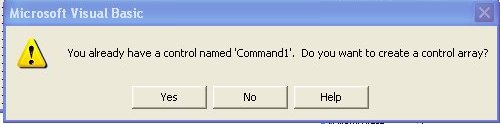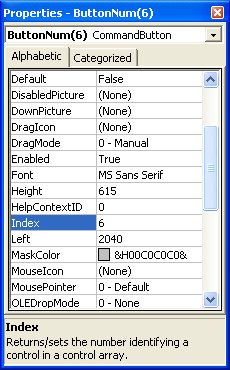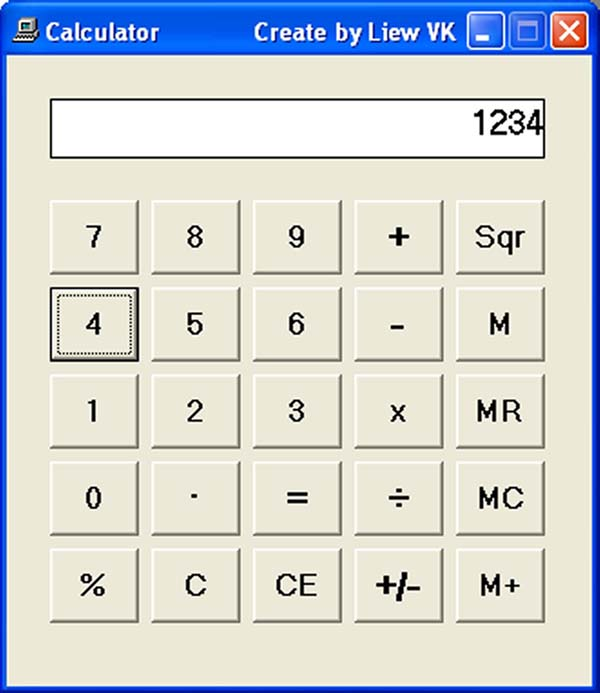# Visual Basic Sample Code

This is a typical calculator that consists of the number buttons, the operator buttons and some additional buttons such as the memory button and the clear button. To design the interface, you need to insert 25 command buttons, and one label that functions as the display panel. Name the display panel simply as panel. The number buttons from 1 to 9 are grouped together as a control array and named as ButtonNum while 0 is a standalone command and named as Bzero. The simplest way to create the control array is to insert a command button then right click to copy it then click paste, you will be presented with a pop-up dialog as shown below:Click yes and a new command button will appear. Repeat the process nine times and you will get all the nine buttons in the array. We name the control array ButtonNum as each number is differentiated by its index. The nine button's names are ButtonNum(1), ButtonNum(2),ButtonNum(3)............,ButtonNum(9).

The four basic operators are also grouped together as a control array and named as Operator. Other buttons are named appropriately according to their functions. The label is named as panel.

One of the most important procedures in the program is to display the number on the panel. To display the number on the panel, click on any number button and enter the following code, as shown below:

Private Sub ButtonNum_Click(Index As Integer)

If Num_of_digit > 0 Then

If Num_of_digit < 30 Then

panel. Caption = panel.Caption + Right\$(Str(Index), 1)

Num_of_digit = Num_of_digit + 1

End If

Else

panel.Caption = Right\$(Str(Index), 1)

Num_of_digit = 1

End If>

CheckValue

End Sub

The Num_of_digit is a variable that is used to check the number of digits that appear on the display panel. The procedure will ensure that if the number of digits is more than one, the preceding digit will be pushed to the left and the succeeding digit will remain on the right. However, if the number of digits is zero, the digit clicked will just appear on the rightmost position of the panel.

The index of a particular  number button is displayed on the display panel using the syntax Right\$(Str(Index), 1). Make sure you label the number button with a number that corresponds with the index of the button. For example, you must label ButtonNum(6) with the number 6 so as it corresponds to its index, as shown in the Figure on the right.

The subroutine CheckValue is to assign the value displayed on the panel to the variable displayValue. This value can then be manipulated using any of  the arithmetic operators.

We introduce the variables a,b,c,d,e,f to accept the value of the first number entered by the user and we use the variable key to determine what arithmetic operation to be carried out when the Equal button is pressed. We also use the boolean newNumber to determine the number entered is new or not.## The Code

Sub CheckValue( )

displayValue = Val(panel.Caption)

End Sub

'The code for the operators is as follow:

Private Sub Operator_Click(Index As Integer)

CheckValue
If Index = 11 Then
a = displayValue
key = 1

ElseIf Index = 12 Then
b = displayValue
key = 2

ElseIf Index = 13 Then
c = displayValue
key = 3

ElseIf Index = 14 Then
d = displayValue
key = 4

ElseIf Index = 15 Then
f = displayValue
key = 5

End If

Num_of_digit = 0
newNumber = True

End Sub

'The code for the Equal button is as follow:

Private Sub Equal_Click()

CheckValue
If newNumber = True Then

If key = 1 Then
e = displayValue + a
ElseIf key = 2 Then
e = b - displayValue

ElseIf key = 3 Then
e = displayValue * c

ElseIf key = 5 Then
e = (f * displayValue) / 100

ElseIf key = 4 And displayValue <> 0 Then
e = d / displayValue

Else

GoTo error

End If
If Abs(e) < 1 Then
panel.Caption = Format(e, "General Number")
Else

panel.Caption = Str(e)
End If
Else
panel.Caption = displayValue

End If

GoTo finish

error: panel.Caption = "E"

finish:

Num_of_digit = 0
newNumber = False

End Sub

### The Interface